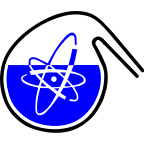#RadChem 2018

13-18 May 2018
Casino Conference Centre
Europe/Prague timezone

## Thermodynamics of the complexation of neptunium (V) with halides in aqueous solution at elevated temperatures

15 May 2018, 09:30
15m
Red Hall (Casino Conference Centre)

### Red Hall

#### Casino Conference Centre

Reitenbergerova 4/95, Mariánské Lázně, Czech Republic
Verbal Chemistry of Actinide and Trans-actinide Elements

### Speaker

Mr Martin M. Maiwald (Ruprecht-Karls Universität Heidelberg)

### Description

Introduction
Deep geological salt rock formations are in discussion to serve as host rock for a repository of high-level nuclear waste. This approach requires a well-founded knowledge of the geochemical behaviour of the radionuclides in diluted to concentrated salt solutions to model their potential migration and retention in the near- and far-field of the repository over long time scales. In the past a broad variety of complexation reactions of actinides in their most important oxidation states were studied. The thermodynamic functions (log $\beta^{0}_j$, $\Delta_{r}H^{0}_m$, $\Delta_{r}S^{0}_m$, $\Delta_{r}C^{0}_{p,m}$) and modelling parameters ($\epsilon_{T}(i,k)$) are summarized in the NEA thermodynamic database.$^{1}$ However, most data are only available for 25 °C or fixed ionic strength.$^{2a,b}$ Temperatures up to 200 °C are expected in the near-field of a repository for high-level nuclear waste, which will significantly alter the thermodynamics of the geochemical reactions of the radionuclides in solution. Thus, detailed thermodynamic data at 25°C as well as at increased temperatures are mandatory for a comprehensive long-term safety assessment.

Results and Discussion
In this work the complexation of NpO$_2$$^{+}$ with F$^-$ and Cl$^-$ was studied as a function of the temperature (20 – 85 °C) and the ionic strength (I$_m$(NaClO$_{4}$) = 0.5 – 4.0 mol kg$^{-1}$) using near infrared (NIR) absorption spectroscopy. Systematic evaluation of the spectroscopic results clearly confirms the formation of two distinct complex species [NpO$_2$(L)$_2$]$^{1-n}$ (n = 1, 2) for L = F$^{-}$ and Cl$^{-}$. Applying the specific ion interaction theory (SIT) and the integrated Van´t Hoff equation the thermodynamic functions (log $\beta^{0}_j$, $\Delta_{r}H^{0}_m$, $\Delta_{r}S^{0}_m$) and the binary ion-ion-interaction parameters ($\epsilon_{T}(i,k)$) for the different complexation reactions are obtained. With increasing temperature the chemical equilibrium shifts towards the complexed Np(V) species for both ligands. The thermodynamic stability constants increase by 0.5 - 1 orders of magnitude with increasing temperature. Furthermore, all complexation reactions show positive values of $\Delta_{r}H^{0}_m$ and $\Delta_{r}S^{0}_m$. Thus, the reactions are endothermic and driven by the high gain of entropy.

References
1. Guillaumont, R et al., Update on the Chemical Thermodynamics of Uranium, Neptunium, Plutonium, Americium and Technetium. Elsvier B.V.: 2003.
2. a) Xia, Y. et al., J. Radioanal. Nucl. Chem. 2006, 268 (3), 445-451 b) Neck, V., et al., Radiochimica Acta 1995, 69(1), 39-47

### Primary author

Mr Martin M. Maiwald (Ruprecht-Karls Universität Heidelberg)

### Co-authors

Dr David Fellhauer (Institute for Nuclear Waste Disposal) Dr Andrej Skerencak-Frech (Institute for Nuclear Waste Disposal) Prof. Petra J. Panak (Ruprecht-Karls Universität Heidelberg)

### Presentation Materials

There are no materials yet.